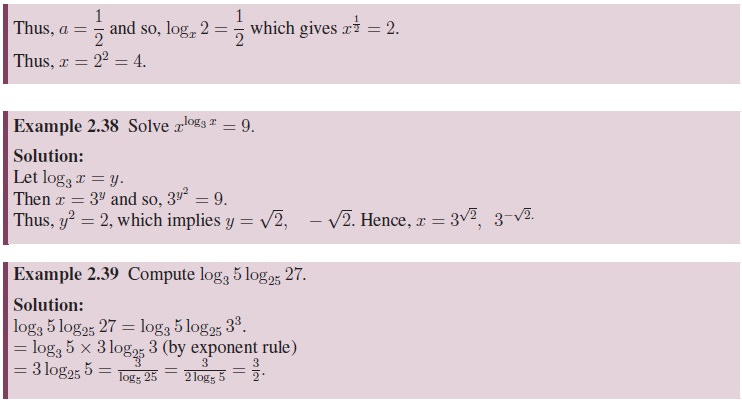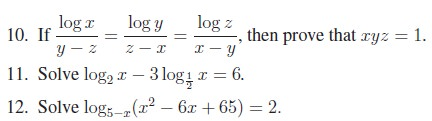Home | | Maths 11th std | Logarithm

# Logarithm

We also observed that f(x) is a bijection, hence it has an inverse. We call this inverse function as logarithmic function and is denoted by loga(.).

Logarithm

We have seen that, with a base 0 < a ¹ 1, the exponential function f(x) = ax is defined on R having range (0, ). We also observed that f(x) is a bijection, hence it has an inverse. We call this inverse function as logarithmic function and is denoted by loga(.). Let us discuss this function further. Note that if f(x) takes x to y = ax, then loga(.) takes y to x. That is, for 0 < a ¹ 1, we have

y = ax is equivalent to loga y = x.

For example, since 34 = 81 we have log3(81) = 4. In other words, with fixed a, given a real number y, logarithm finds the exponent x satisfying ax = y. This is useful in addressing practical problems like, “how long will it take for certain investment to reach a fixed amount?” Logarithm is also very useful in multiplying very small or big numbers.## Properties of LogarithmTags : Definition, Properties, Proof, Solved Example Problems, Exercise | Mathematics , 11th Mathematics : UNIT 2 : Basic Algebra
Study Material, Lecturing Notes, Assignment, Reference, Wiki description explanation, brief detail
11th Mathematics : UNIT 2 : Basic Algebra : Logarithm | Definition, Properties, Proof, Solved Example Problems, Exercise | Mathematics